# 深度学习项目实战——“年龄预测”

+关注继续查看

• 花时间建立基础：我一直建议，我们应该始终建立一个正确的基础（思考问题陈述和探索数据集），并练习很多。但我仍然看到人们一开始就编码，而不考虑问题和理解数据。在这种方法中，你实际上不是在探索问题和数据，因为你正在专注于算法。
• 同行学习（论坛/博客）：在实践中，参与者在论坛或博客上分享他们的方法，并随时准备讨论新的方法。这实际上就是一种开源的学习方式。
• 练习：这些实践问题就像你的练习课，在出山和解决现实生活中的问题之前。你应该首先多多练习，然后评估你的表现。相信熟能生巧，这句话。
• 测试你的知识：这是一个伟大的方式，尝试练习你学到的东西，这将是非常有益。结果并不重要，因为这是一个练习问题。

% pylab inline
import os
import random
import pandas as pd
from scipy.misc import imread

root_dir = os.path.abspath('.')
data_dir = '/mnt/hdd/datasets/misc'
test = pd.read_csv(os.path.join(data_dir, 'test.csv'))

i = random.choice(train.index)
img_name = train.ID[i]
imshow(img)
print(‘Age: ‘, train.Class[i])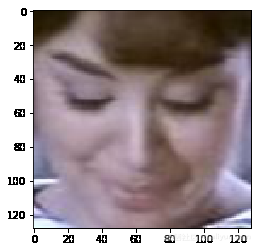1.形状变化：一个图像是（66,46），而另一个图像是（102,87）。

2.多个方向：我们的图像可能是多个方向的，这里有些例子：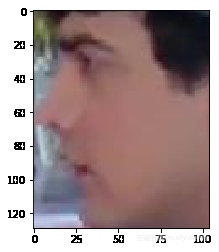3.图像质量：一些图像的质量可以太差了点，例如下面这张：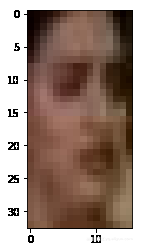4.亮度和对比度差异：检查下面的图像， 他们似乎是故意来捣乱的，但是，这种现象在实际生活中确实存在。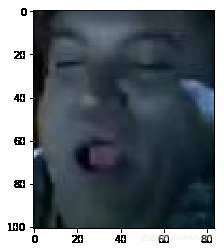from scipy.misc import imresize
temp = []
for img_name in train.ID:
img_path = os.path.join(data_dir, 'Train', img_name)
img = imresize(img, (32, 32))
img = img.astype('float32') # this will help us in later stage
temp.append(img)
train_x = np.stack(temp)

temp = []
for img_name in test.ID：
img_path = os.path.join（data_dir，'Test'，img_name）
img = imresize（img，（32,32））
temp.append（img.astype（ 'FLOAT32'））
test_x = np.stack（temp）

train_x = train_x / 255.
test_x = test_x / 255.

train.Class.value_counts(normalize=True)
MIDDLE    0.542751
YOUNG     0.336883
OLD       0.120366
Name: Class, dtype: float64

test['Class'] = 'MIDDLE'
test.to_csv(‘sub01.csv’, index=False)

## 让我们解决问题！第2部分：建立更好的模型

import keras
from sklearn.preprocessing import LabelEncoder
lb = LabelEncoder()
train_y = lb.fit_transform(train.Class)
train_y = keras.utils.np_utils.to_categorical(train_y)

input_num_units = (32,32,3)
hidden_num_units = 500
output_num_units = 3
epochs = 5
batch_size = 128

from keras.models import Sequential
from keras.layers import Dense, Flatten, InputLayer

model = Sequential([
InputLayer(input_shape=input_num_units),
Flatten(),
Dense(units=hidden_num_units, activation='relu'),
Dense(units=output_num_units, activation='softmax'),
])

model.summary()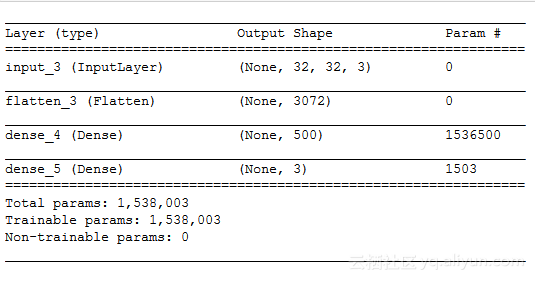model.compile(optimizer='sgd', loss='categorical_crossentropy', metrics=['accuracy'])
model.fit(train_x, train_y, batch_size=batch_size,epochs=epochs,verbose=1)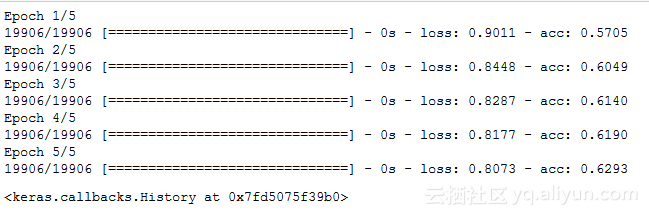model.fit（train_x，train_y，batch_size = batch_size，epochs = epochs，verbose = 1，validation_split = 0.2）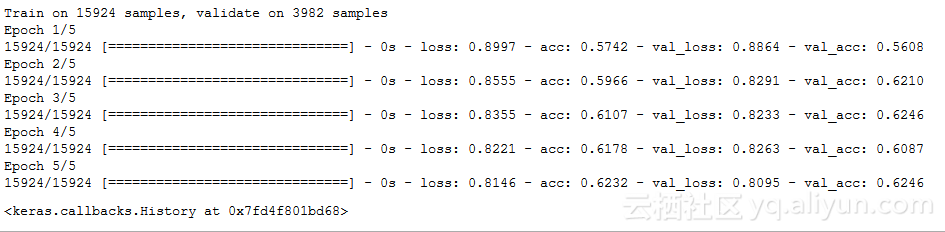pred = model.predict_classes（test_x）
pred = lb.inverse_transform（pred）
test ['Class'] = pred
test.to_csv（'sub02.csv'，index = False）

## 实际检查我们的预测（实际检验）

i = random.choice(train.index)
img_name = train.ID[i]
imshow(imresize(img, (128, 128)))
pred = model.predict_classes(train_x)
print('Original:', train.Class[i], 'Predicted:', lb.inverse_transform(pred[i]))
Original: MIDDLE Predicted: MIDDLE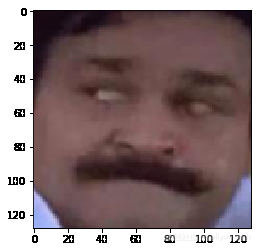• 一个好的神经网络模型可以帮你取得更大的进步。你可以尝试使用更适合图像相关问题的卷积神经网络。这是一个简单的CNN供你参考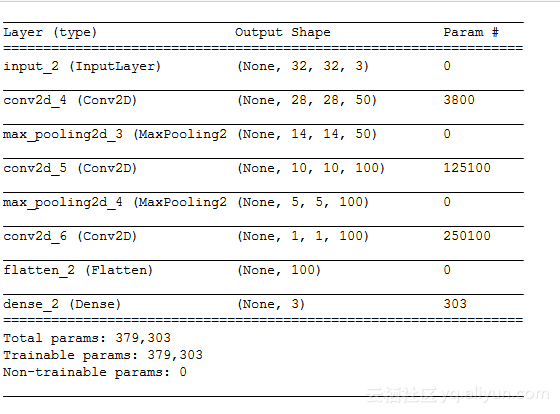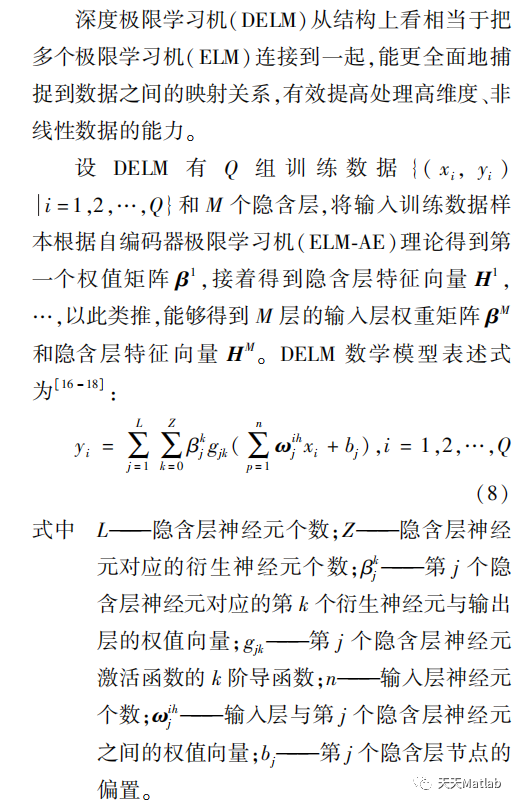【DELM回归预测】基于海鸥算法改进深度学习极限学习机SOA-DELM实现数据回归预测附matlab代码
【DELM回归预测】基于海鸥算法改进深度学习极限学习机SOA-DELM实现数据回归预测附matlab代码
0 0【DELM回归预测】基于粒子群算法改进深度学习极限学习机PSO-DELM实现数据回归预测附matlab代码
【DELM回归预测】基于粒子群算法改进深度学习极限学习机PSO-DELM实现数据回归预测附matlab代码
0 0【DELM回归预测】基于灰狼算法改进深度学习极限学习机GWO-DELM实现数据回归预测附matlab代码
【DELM回归预测】基于灰狼算法改进深度学习极限学习机GWO-DELM实现数据回归预测附matlab代码
0 0【DELM回归预测】基于人工蜂群算法改进深度学习极限学习机ABC-DELM实现数据回归预测附matlab代码
【DELM回归预测】基于人工蜂群算法改进深度学习极限学习机ABC-DELM实现数据回归预测附matlab代码
0 0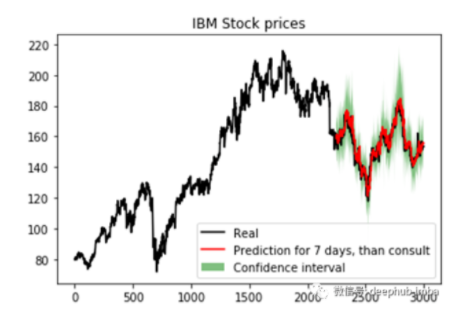Pytorch贝叶斯深度学习库BLiTZ实现LSTM预测时序数据（二）
Pytorch贝叶斯深度学习库BLiTZ实现LSTM预测时序数据（二）
0 0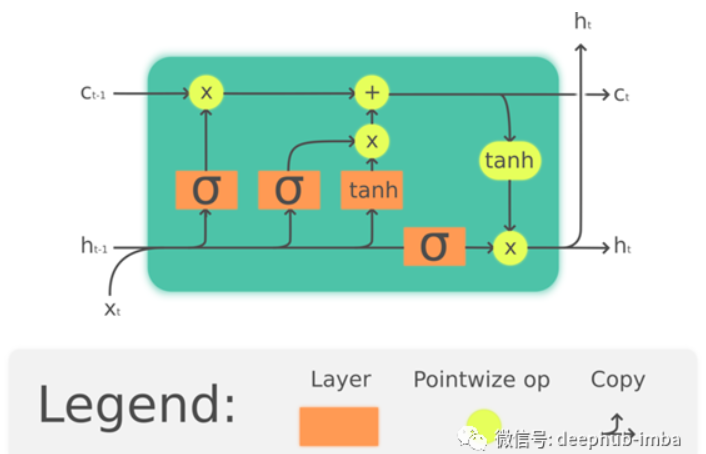Pytorch贝叶斯深度学习库BLiTZ实现LSTM预测时序数据（一）
Pytorch贝叶斯深度学习库BLiTZ实现LSTM预测时序数据（一）
0 0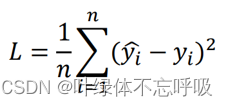0 0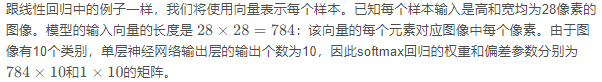【从零开始学习深度学习】7.自己动手实现softmax回归的训练与预测
【从零开始学习深度学习】7.自己动手实现softmax回归的训练与预测
0 0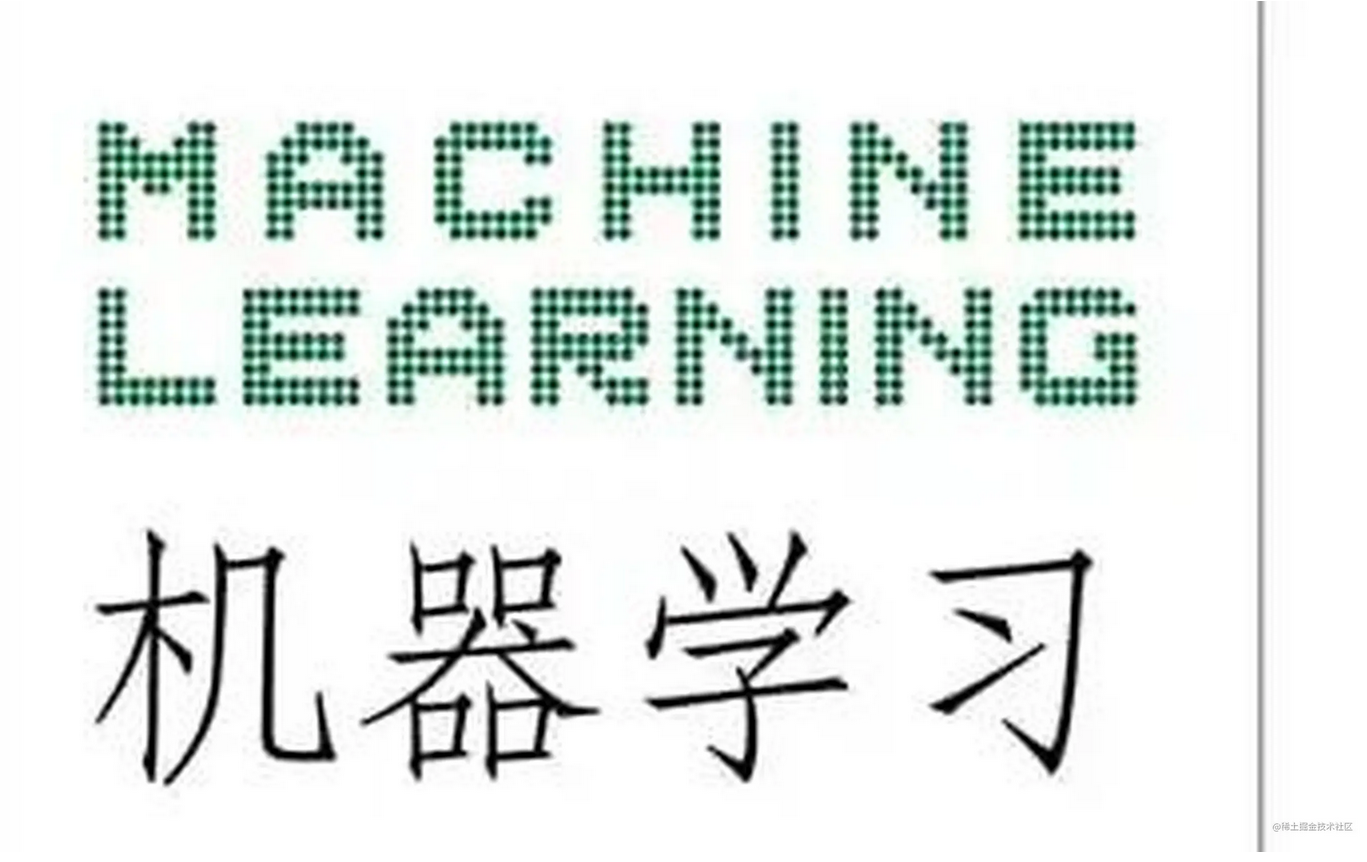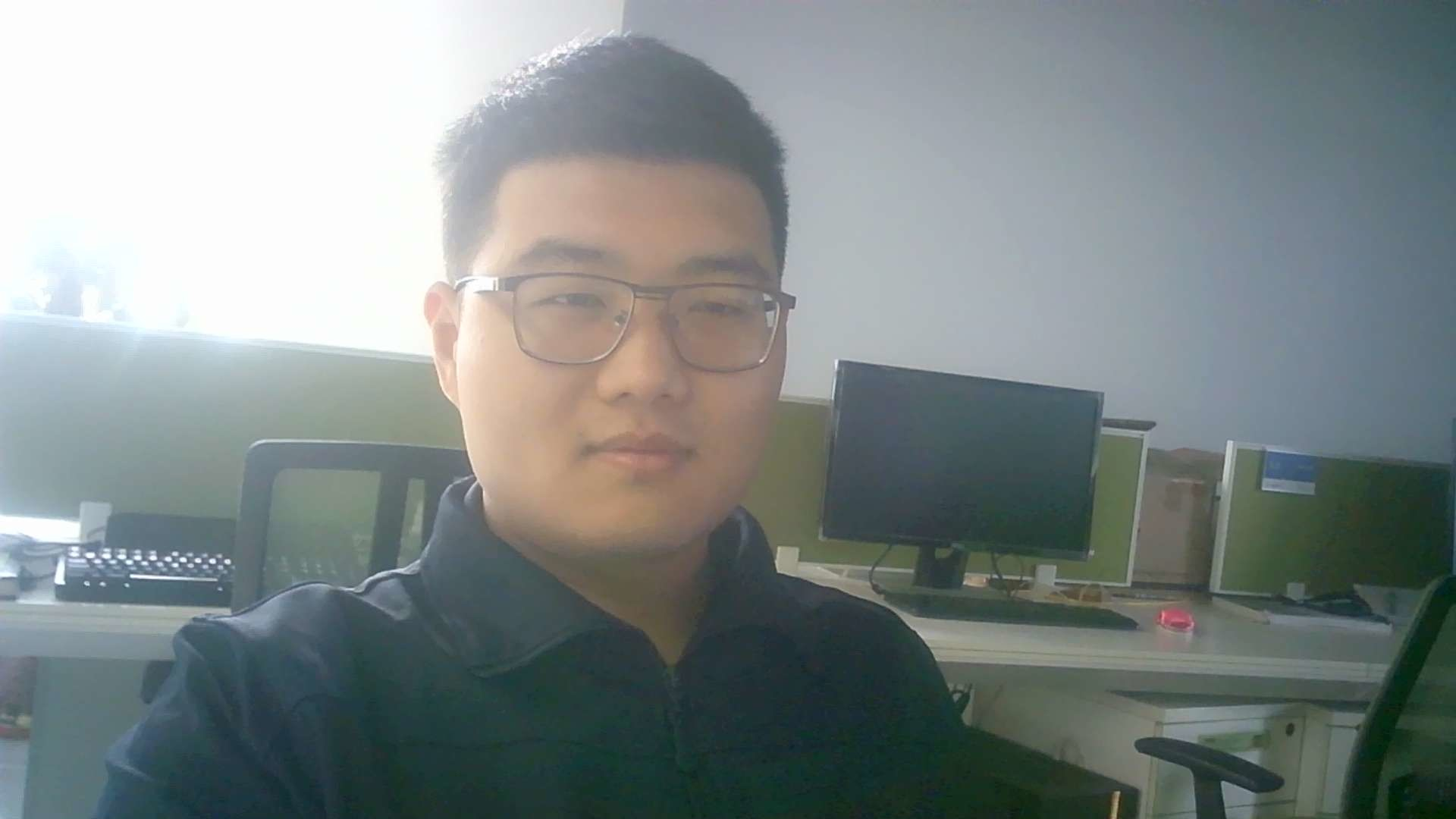0 0【回归预测-DELM】基于布谷鸟算法改进深度学习极限学习机实现数据回归预测附matlab代码
【回归预测-DELM】基于布谷鸟算法改进深度学习极限学习机实现数据回归预测附matlab代码
0 0527

【机器学习】机器学习笔试题集合19576642488

【算法实战】1. 机器学习基础140327517